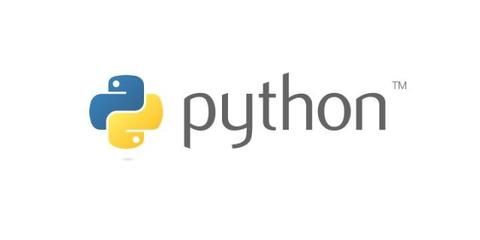# MongoDB 流式聚合(管道与表达式) - 知乎

01/26 09:07

## MongoDB流式聚合

MongoDB中聚合(aggregate)主要用于处理数据(诸如统计平均值,求和等)，并返回计算后的数据结果。有点类似sql语句中的 count(*)。

## aggregate() 方法

MongoDB中聚合的方法使用aggregate()。

### （1）语法

aggregate() 方法的基本语法格式如下所示：

>db.COLLECTION_NAME.aggregate(AGGREGATE_OPERATION)

$sum 计算总和。 db.mycol.aggregate([{$group : {_id : "$by_user", num_tutorial : {$sum : "$likes"}}}])$avg 计算平均值 db.mycol.aggregate([{$group : {_id : "$by_user", num_tutorial : {$avg : "$likes"}}}])
$min 获取集合中所有文档对应值得最小值。 db.mycol.aggregate([{$group : {_id : "$by_user", num_tutorial : {$min : "$likes"}}}])$max 获取集合中所有文档对应值得最大值。 db.mycol.aggregate([{$group : {_id : "$by_user", num_tutorial : {$max : "$likes"}}}])
$push 在结果文档中插入值到一个数组中。 db.mycol.aggregate([{$group : {_id : "$by_user", url : {$push: "$url"}}}])$addToSet 在结果文档中插入值到一个数组中，但不创建副本。 db.mycol.aggregate([{$group : {_id : "$by_user", url : {$addToSet : "$url"}}}])
$first 根据资源文档的排序获取第一个文档数据。 db.mycol.aggregate([{$group : {_id : "$by_user", first_url : {$first : "$url"}}}])$last 根据资源文档的排序获取最后一个文档数据 db.mycol.aggregate([{$group : {_id : "$by_user", last_url : {$last : "$url"}}}])

（2）概念

MongoDB的聚合管道将MongoDB文档在一个管道处理完毕后将结果传递给下一个管道处理。管道操作是可以重复的。

• $project：修改输入文档的结构。可以用来重命名、增加或删除域，也可以用于创建计算结果以及嵌套文档。 •$match：用于过滤数据，只输出符合条件的文档。$match使用MongoDB的标准查询操作。 •$limit：用来限制MongoDB聚合管道返回的文档数。
• $skip：在聚合管道中跳过指定数量的文档，并返回余下的文档。 •$unwind：将文档中的某一个数组类型字段拆分成多条，每条包含数组中的一个值。
• $group：将集合中的文档分组，可用于统计结果。 •$sort：将输入文档排序后输出。
• $geoNear：输出接近某一地理位置的有序文档。 （3）查询条件 1 ) 大于，小于，大于或等于，小于或等于$gt:大于
$lt:小于$gte:大于或等于
$lte:小于或等于 例子： db.collection.find({ "field" : {$gt: value } } ); // greater than : field > value
db.collection.find({ "field" : { $lt: value } } ); // less than : field < value db.collection.find({ "field" : {$gte: value } } ); // greater than or equal to : field >= value
db.collection.find({ "field" : { $lte: value } } ); // less than or equal to : field <= value如查询j大于3,小于4: db.things.find({j : {$lt: 3}});
db.things.find({j : {$gte: 4}});也可以合并在一条语句内: db.collection.find({ "field" : {$gt: value1, $lt: value2 } } ); // value1 < field < value 2) 不等于$ne

db.things.find( { x : { $ne : 3 } } ); 3) in 和 not in ($in $nin) 语法： db.collection.find( { "field" : {$in : array } } );例子：
db.things.find({j:{$in: [2,4,6]}}); db.things.find({j:{$nin: [2,4,6]}});

4) 取模运算$mod 如下面的运算： db.things.find( "this.a % 10 == 1")可用$mod代替：
db.things.find( { a : { $mod : [ 10 , 1 ] } } ) 5)$all

$all和$in类似，但是他需要匹配条件内所有的值：

{ a: [ 1, 2, 3 ] }下面这个条件是可以匹配的：
db.things.find( { a: { $all: [ 2, 3 ] } } );但是下面这个条件就不行了： db.things.find( { a: {$all: [ 2, 3, 4 ] } } );

6) $size$size是匹配数组内的元素数量的，如有一个对象：{ a:["foo"] }，他只有一个元素：

You cannot use $size to find a range of sizes (for example: arrays with more than 1 element). If you need to query for a range, create an extra size field that you increment when you add elements. 7）$exists
$exists用来判断一个元素是否存在： 如： db.things.find( { a : {$exists : true } } ); // 如果存在元素a,就返回
db.things.find( { a : { $exists : false } } ); // 如果不存在元素a，就返回 8)$type

$type 基于 bson type来匹配一个元素的类型，像是按照类型ID来匹配，不过我没找到bson类型和id对照表。 db.things.find( { a : {$type : 2 } } ); // matches if a is a string
db.things.find( { a : { $type : 16 } } ); // matches if a is an int 9）正则表达式 mongo支持正则表达式，如： db.customers.find( { name : /acme.*corp/i } ); // 后面的i的意思是区分大小写 10) 查询数据内的值 下面的查询是查询colors内red的记录，如果colors元素是一个数据,数据库将遍历这个数组的元素来查询。db.things.find( { colors : "red" } ); 11)$elemMatch

$elemMatch是匹配{ "a" : 1, "b" : 3 }，而后面一句是匹配{ "b" : 99 }, { "a" : 11 } 12) 查询嵌入对象的值 db.postings.find( { "author.name" : "joe" } );注意用法是author.name，用一个点就行了。更详细的可以看这个链接： dot notation 举个例子： > db.blog.save({ title : "My First Post", author: {name : "Jane", id : 1}})如果我们要查询 authors name 是Jane的, 我们可以这样： > db.blog.findOne({"author.name" : "Jane"})如果不用点，那就需要用下面这句才能匹配： db.blog.findOne({"author" : {"name" : "Jane", "id" : 1}})下面这句： db.blog.findOne({"author" : {"name" : "Jane"}})是不能匹配的，因为mongodb对于子对象，他是精确匹配。 13) 元操作符$not 取反

db.customers.find( { name : { $not : /acme.*corp/i } } );db.things.find( { a : {$not : { \$mod : [ 10 , 1 ] } } } ); mongodb还有很多函数可以用， 如排序，统计等。

## 结论

pandas耗时：

mongoDB耗时：

yeayee：Python数据分析及可视化实例目录zhuanlan.zhihu.com0
0 收藏

### 作者的其它热门文章0 评论
0 收藏
0